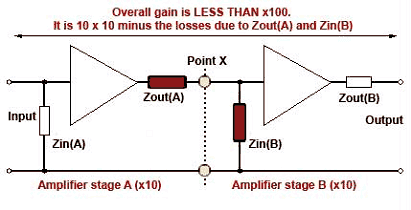# Impedance Matching.

• After studying this section, you should be able to describe:
• •Impedance matching
• •The effects of input and output impedance on amplifier gain### Fig 7.2.1 Defining the Impedance Frequency.

The component or circuit will not have the same impedance at all frequencies. It is common for inputs and outputs on many types of equipment to have their impedances quoted in Ohms and to assume a common frequency for that particular type of equipment. For example, audio commonly uses a frequency of 1kHz as the standard for measuring impedance. This is because 1kHz is approximately the centre of an audio amplifier´s bandwidth, measured on a logarithmic scale, as shown in Fig 7.2.1. The thing to notice in equipment designed to interconnect with other units is that most inputs (to amplifiers etc) have high impedances (in Ohms) whereas outputs have much lower impedances. The reason for this is because of the effects of impedance.### Fig 7.2.2 Input and Output Impedances

An amplifier can be considered to have two impedances that affect the way it can be connected to other amplifiers, one impedance is considered to be connected across the input terminals (because when an input source is connected to these terminals, a current flows across the terminals, therefore, there must be an impedance (shown as a resistor) for the current to flow through. At the output there is another imaginary resistor (impedance), this time connected in series with the flow of current. The effect of this output impedance is to reduce the voltage at the output terminals, and the more current drawn from the output, the more the terminal voltage is reduced, therefore there must be an (invisible) resistance across which the voltage is being dropped. The input and output impedances of an amplifier are illustrated in Fig 7.2.2### Fig. 7.2.3 Matching Input & Output Impedances.

Impedance matching of inputs and outputs is necessary because the gain of a single amplifier is often insufficient for a given purpose. For this reason several stages of amplification are used, which involves feeding the output of one amplifier into the input of another. This is called connecting the amplifiers in "Cascade". It is then important that the output impedance of the first amplifier and the input impedance of the second amplifier, which effectively form a potential divider as shown in Fig 7.2.3 do not unduly reduce the overall gain of the cascaded amplifiers.

## Matching Voltage Amplifiers

If the amplifiers are voltage amplifiers, i.e. amplifier stages whose voltage gain is greater than either their current or power gains, the voltage waveform needs to be coupled from one stage to the next with as little reduction in its voltage amplitude as possible. Notice that the output impedance of the first stage and the input impedance of the second stage form a potential divider, as shown in the shaded portion of Fig. 7.2.3. The voltage available at the junction of the two impedances will depend on the relative values of Zin (B) to Zout (A). The higher the value of an impedance, the more voltage will be developed across it for a given current flow. Provided that Zout (A) is much smaller than Zin (B), the majority of the available voltage at point X (the input to stage B) will be developed across its input impedance Zin (B), and so across stage B input terminals rather than across the first amplifier´s output impedance.

## Matching Power Amplifiers

In the case of power amplifiers however, as the waveform current is shared between the input and output impedances in opposite proportions the waveform voltage, the maximum power (V x I) is transferred from output to input if both impedances are equal.

The values of input and output impedance therefore have a considerable effect on the gain of multi stage amplifiers. There is always some loss of signal amplitude that occurs due to the coupling of successive amplifier stages, and in calculating the overall gain of a multi stage amplifier, the overall gain will be less than equal to the product of the individual gains of each amplifier. I.e if each stage of a two stage amplifier has a gain of 10, then the overall gain might be supposed to be 10 x 10 = 100; in practice however, this is not achievable due to the coupling losses incurred in matching the amplifiers, and because of even the best impedance matching, a slightly lower gain results. The value of input and output impedance can be arranged to an appropriate value by the choice of amplifier design. More complex methods of controlling impedance in multi-stage amplifiers, using negative feedback, is dicussed in Amplifiers Module 3.2.

Although the above description refers to coupling amplifiers together, the same considerations apply when an amplifier is driving a power device such as a loudspeaker, a current operated device such as a motor, or in matching an input device such as a microphone to the input of a voltage amplifier.